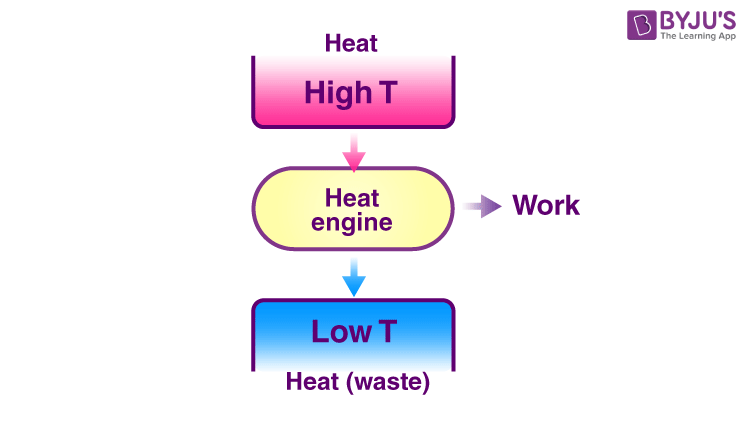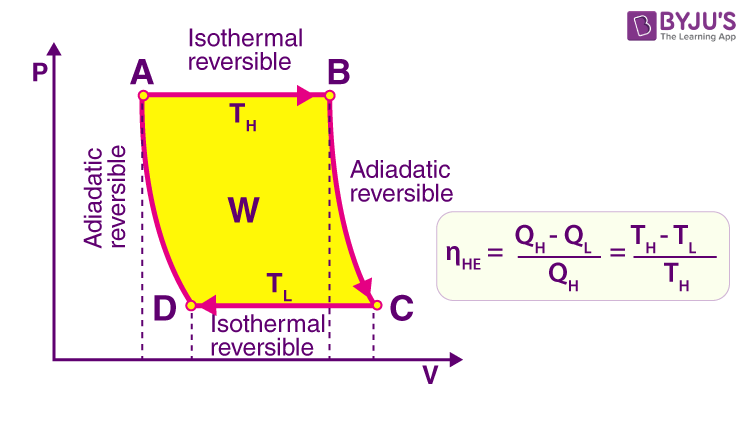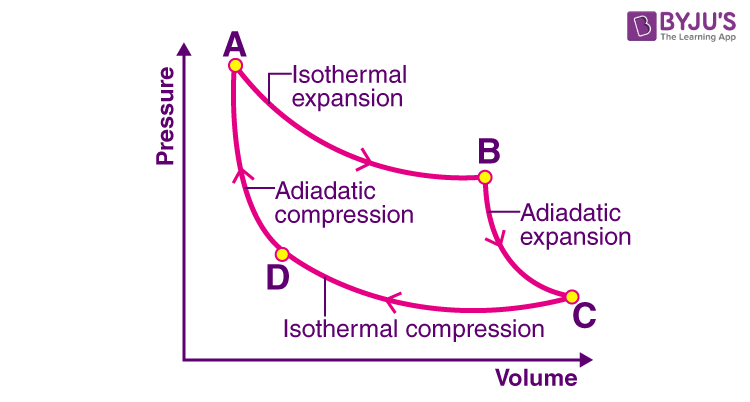Know more about the Repeaters Batch Know more about the Repeaters Batch

# Heat Engine

A heat engine is a system which converts heat into work by taking heat from the reservoir ( hot body) to carry out some work. There is a discharge of some heat to the sink (cold body). In this system, there will also be some waste in the form of heat. There are different types of a heat engine in which a Carnot engine has the maximum efficiency.

A very basic diagram of the heat engine is given below:We know that thermodynamics is the study of the relation between heat and work. The first law of thermodynamics and the second law of thermodynamics helps in the operation of the heat engine.

The first law is the application of conservation of energy and the second law sets limits on the possible efficiency of the machine and determines the direction of flow of energy.

## Types of Heat Engine

There are mainly two types of heat engines – external combustion engine and internal combustion engine.

• External Combustion Engine

Here the fuel gets burned outside the engine or at a far distance from the engine by which it can produce force and motion. A very good example of an external combustion engine is a steam engine.

Water is heated outside or far away from the system by using coal fire and the steam produced is given to the top of the metal cylinder through pipes and the heat is given to the engine and it moves the piston back and forth and the work is done. The fluid is then cooled, compressed and reused.

• Internal combustion engine

Here the fuels are burned inside the champer. Car engine a very good example of this type of combustion engine.

The heat produced due to combustion is given to the engine and the work is done. It is also called 4-stroke because it takes 4 strokes for the piston to complete one combustion cycle.

So, if we consider the two types of heat engine, Internal combustion engines are more efficient than external combustion engine. This is because no energy is wasted in an internal combustion engine by transferring heat from the boiler to the cylinder as in an external combustion engine. Everything happens in one place.

## Working of Heat Engine

As we look earlier, a heat engine contains basically a heat reservoir, engine and a cold sink. The heat that we produce as internal combustion or external combustion is given to the engine where the movement of the piston takes place. The power generated is given to the machine that connected to the engine and hence the work is done. Excess heat is given to the sink where the temperature will remain the same at both reservoir and sink.

Heat engine like automobile engines operated in a cyclic manner. They will add energy in the form of heat in one part of the cycle and use this energy to do useful work from another part of the cycle.

## Heat Engine PV Diagram

Heat engines can be typically illustrated on a PV diagram,Pressure-Volume (PV) diagrams are the basic tool for the study of heat engines that use gas as the working substance.PV diagram will be a closed-loop for a cyclic heat engine. The area of the loop is the representation of the amount of work done during the cycle.

The idea of the efficiency of an engine cycle can be obtained by comparing the PV diagram with the Carnot cycle, which is the most efficient type of heat engine.

Explanation of PV diagram:

• The fluid changes from liquid to vapour isothermally if the source is at a high temperature. This vaporization process occurs at constant pressure and increasing volume.
• At the turbine end, the gas expands reversibly and adiabatically and it follows the equation of state for an adiabatic and reversible process.
• The fluid changes from a gas to liquid isothermally if the source is at a low temperature. This condensation process occurs at constant pressure and decreasing volume.
• At the compressor end, the liquid is compressed reversibly and adiabatically by increasing its pressure to the original point.

## Heat Engine Efficiency

The efficiency is the fraction of heat input at high-temperature changes to work. In the second law of thermodynamics, it says that no engine is 100% efficient.

Efficiency, η = Work done / Heat input

We know that,

Work done, W = Q1 – Q2

Heat input = Q1

Then,

Efficiency, η = W / Q1

= (Q1 – Q2) / Q1

=1 – (Q2 / Q1)

## Carnot Engine

A Carnot engine is an ideal engine that can operate in a reversible closed thermodynamic cycle where the working substance goes through four successive operations such as isothermal expansion, adiabatic expansion, isothermal compression and adiabatic compression. It is the basis of other thermodynamic cycles that have vast applications in industries. Two common applications are the Carnot heat engine and refrigerators.

Although the Carnot engine is the ideal engine, in reality, no engine achieves Carnot’s theoretical maximum efficiency since friction plays an important role. The efficiency of a Carnot engine uniquely depends on the temperature of the hot and cold reservoir.

Carnot engine basically draws heat (Q1) from the source and rejects heat (Q2) to the sink while performing an amount of work, W = Q1 – Q2.The complete cycle incorporates:

• Isothermal Expansion: During the isothermal expansion of an ideal gas we use the temperature of source T1 which will draw Q1 amount of heat. Since the expansion is isothermal the total change in internal energy is zero and the heat absorbed by the gas will be equal to the work done by the gas on the environment.
• Adiabatic Expansion: During the adiabatic expansion of an ideal gas, the temperature of an ideal gas falls from source temperature T1 to sink temperature T2. Here, the pressure falls and volume increases because no heat energy is being supplied to gas as it expands. The work is less and the pressure must be lower.
• Isothermal Compression: During the process of isothermal compression of an ideal gas sink temperature T2 further goes down as it rejects heat Q2 to the sink.
• Adiabatic Compression: During adiabatic compression of an ideal gas temperature naturally raises from T2 to T1 and hence working substance returns to its original state by completing one cycle.

The efficiency of the Carnot engine is given as,

η =Work done / Heat input

We know that,

Work done, W = Q1 -Q2

Heat input =Q1

Then,

Efficiency, η =W / Q1

=(Q1-Q2) /Q1

=1-(Q2 /Q1), which is the efficiency of the heat engine.

If Q2 = 0, then efficiency = 100% . This is known as the Carnot engine.

It is an ideal case where the efficiency is 100%. However, there will be some loss of energy in the system and hence, for every engine, there will be a limit of efficiency.

Meanwhile, it is also known that,

Thermodynamically, (Q2/Q1) = (T2/T1)

Therefore,

η = 1 – (T2/T1)

Work done in each step:

Isothermal expansion:

Wa → b = Q1= n  R T 1 ln(v2 / v1)

Wb → c = ( nR /( γ -1))(T1-T2)

Isothermal compression:

Wc → d = nRT2ln(v3/v4)

Wd → a = ( nR /( γ -1))(T1-T2)

Hence, the total work done by the gas on the environment in one complete cycle is given by, W= Wa → b+Wb → c+Wc → d +Wd → a

Net efficiency = (net work done by the gas) / (heat absorbed by the gas)

=W / Q1

=(Q1-Q2) / Q1

=1-(Q2 / Q1)

## Examples of Different Engines

Steam Engine:

A steam engine is a heat engine that gives mechanical work using steam as working fluid. It uses the force produced by the steam pressure to move the piston forward and backward inside the chamber. This pushing force is transferred to work. This is a type of external combustion engine in which working fluid is burned out of the chamber giving the steam to the source using a pipe.

Car Engine or Gasoline(petrol) Engine:

This is a type of internal combustion engine where combustion takes place internally. The main purpose of a gasoline car engine is to convert gasoline into motion so that your car can move. The easiest way to produce this motion is to burn the gasoline inside the engine. Here the air is also used to burn the working fluid.

Refrigerator or Heat Pump:

A normal refrigerator is an example of a heat pump and in reverse, it is a heat engine. Here, work is used to create a heat differential. Many more cycles can work in reverse to flow heat from the cold side to the hot side. Internal combustion engine parts of these cycle are also not reversible. Heat pump consumes work and is basically used for heating purposes.

Beam Engine:

It is a type of external combustion engines. In olden years, the machine that was used was very gigantic in size almost the size of a room. These early devices had a cylinder and a piston attached to a large beam which performed the working of machines.

Stirling Engines:

Not all external combustion engines are huge and inefficient. Stirling engine consists of two cylinders with piston powering a single wheel. One cylinder is kept permanently hot and the other cylinder is kept permanently cold. The engine works as the movement of gas between the cylinders back and forth.

## Frequently Asked Questions on Heat Engine

### What is a heat engine?

A heat engine is a device that converts heat energy to mechanical energy.

### The efficiency of a heat engine cannot be 100%. Explain why?

The efficiency of a heat engine is given by
ղ = 1 – Q2/Q1
Q2 = heat rejected to the sink
Q1 = heat absorbed from the source
The efficiency of the heat engine will be 100% only when the temperature Q2 is 0. This cannot happen because if Q2 is zero, the temperature of the working substance will go on increasing. At a certain stage, the temperature of the working substance will become equal to the source. There will not be any heat transfer from the source to the working substance in this situation. Hence, we will not get any output.

### A heat engine will convert disordered mechanical motion into ordered mechanical motion. Comment on this statement.

A heat engine is a device which converts heat energy (disordered mechanical motion of the molecules) into kinetic energy (ordered mechanical motion).

### What are the factors on which the efficiency of the Carnot engine depends?

The efficiency of the Carnot’s engine depends on the absolute temperature of the source and sink.

### Why can a ship not use the internal energy of seawater to operate the engine?

If there is a sink at a temperature lower than the temperature of the seawater the heat engine can convert the internal energy of seawater.  Since there is no sink and hence a ship cannot use the internal energy of seawater to operate the engine.

### Heat energy is converted into what form of energy in a heat engine?

Heat energy gets converted into mechanical energy in a heat engine.

Petrol Engine.
Diesel Engine.

### Define the efficiency of the heat engine.

The efficiency of the heat engine is the ratio of mechanical work done to the heat absorbed.

Test Your Knowledge On Heat Engine!